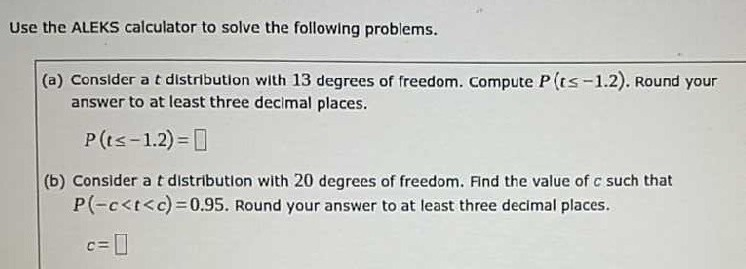### Still have math questions?

Algebra
QuestionUse the ALEKS calculator to solve the following problems.

(a) Consider a $$t$$ distribution with $$13$$ degrees of freedom. Compute $$P ( t \leq - 1.2 )$$. Round your answer to at least three decimal places.

$$P ( t \leq- 1.2)=$$

(b) Consider a $$t$$ distribution with $$20$$ degrees of freedom. Find the value of $$c$$ such that $$P ( - c < t < c ) = 0.95 .$$ Round your answer to at least three decimal places.

$$c = \square$$

(a) $$P(t \le - 1.2) = 0.1258 \approx 0.126$$
(b) $$c= 1.725$$